# GED Math : Angles and Quadrilaterals

## Example Questions

### Example Question #1351 : Ged Math

In Rhombus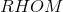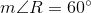. If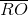is constructed, which of the following is true about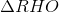?is right and isosceles, but not equilateralis acute and equilateralis obtuse and isosceles, but not equilateralis acute and isosceles, but not equilateralis obtuse and isosceles, but not equilateral

Explanation:

The figure referenced is below.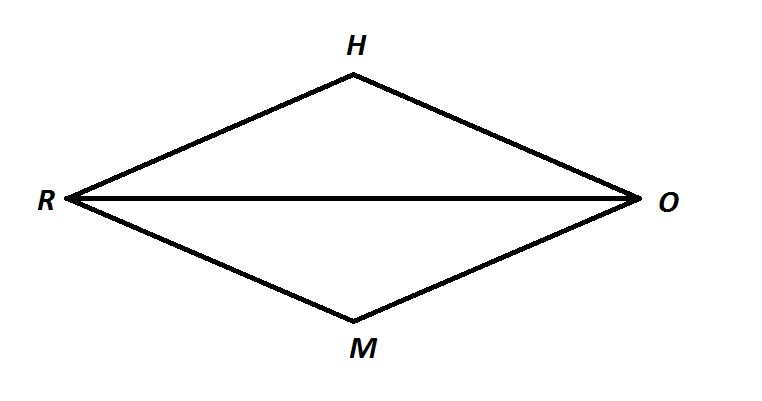The sides of a rhombus are congruent by definition, so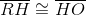, makingisosceles (and possibly equilateral).

Also, consecutive angles of a rhombus are supplementary, as they are with all parallelograms, so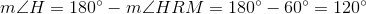.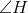, having measure greater than, is obtuse, makingan obtuse triangle. Also, the triangle is not equilateral, since such a triangle must have threeangles.

The correct response is thatis obtuse and isosceles, but not equilateral.

### Example Question #1 : Angles And Quadrilaterals

Given Quadrilateral, which of these statements would prove that it is a parallelogram?

I)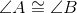and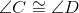II)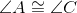and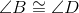III)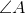and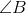are supplementary and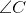and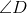are supplementary

Statement III only

Statement I, II, or III

Statement II only

Statement I only

Statement II only

Explanation:

Statement I asserts that two pairs of consecutive angles are congruent. This does not prove that the figure is a parallelogram. For example, an isosceles trapezoid has two pairs of congruent base angles, which are consecutive.

Statement II asserts that both pairs of opposite angles are congruent. By a theorem of geometry, this proves the quadrilateral to be a parallelogram.

Statement III asserts that two pairs of consecutive angles are supplementary. While all parallelograms have this characteristic, trapezoids do as well, so this does not prove the figure a parallelogram.

The correct response is Statement II only.

### Example Question #3 : Angles And Quadrilaterals

You are given Parallelogramwith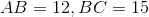. Which of the following statements, along with what you are given, would be enough to prove that Parallelogramis a rectangle?

I)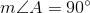II)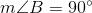III)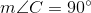Statement III only

Statement I, II, or III

Statement I only

Statement II only

Statement I, II, or III

Explanation:

A rectangle is defined as a parallelogram with four right, or, angles.

Since opposite angles of a paralellogram are congruent, if one angle measures, so does its opposite. Since consecutive angles of a paralellogram are supplementary - that is, their degree measures total- if one angle measures, then both of the neighboring angles measure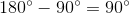.

In short, in a parallelogram, if one angle is right, all are right and the parallelogram is a rectangle. All three statements assert that one angle is right, so from any one, it follows that the figure is a rectangle. The correct response is Statements I, II, or III.

Note that the sidelengths are irrelevant.

### Example Question #4 : Angles And Quadrilaterals

If the rectangle has a width of 5 and a length of 10, what is the area of the rectangle?Explanation:

Write the area for a rectangle.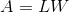Substitute the given dimensions.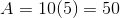The answer is:### Example Question #5 : Angles And Quadrilaterals

In the figure below, find the measure of the largest angle.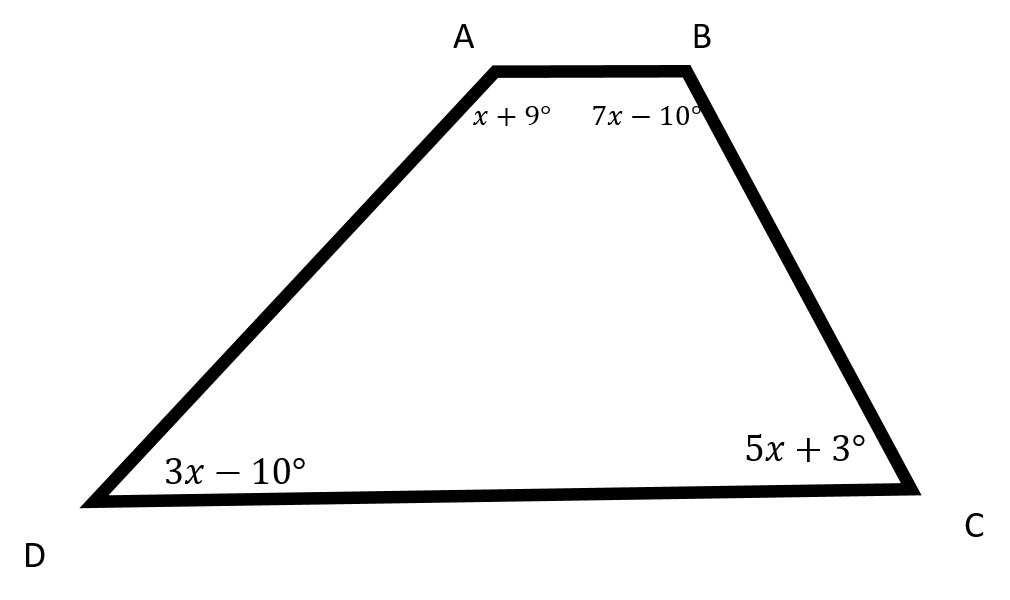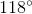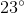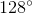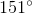Explanation:

Recall that in a quadrilateral, the interior angles must add up to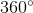.

Thus, we can solve for: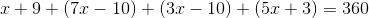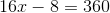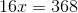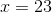Now, to find the largest angle, plug in the value ofinto each expression for each angle.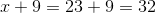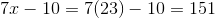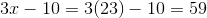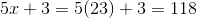The largest angle is.